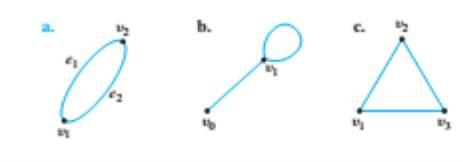Chapter 10.1, Problem 23ES### Discrete Mathematics With Applicat...

5th Edition
EPP + 1 other
ISBN: 9781337694193

#### Solutions

Chapter
Section### Discrete Mathematics With Applicat...

5th Edition
EPP + 1 other
ISBN: 9781337694193
Textbook Problem
1 views

# Find all subgraph of each of the following graphs.To determine

(a)

To find:

All subgraphs of the given graph.

Explanation

Given information:

The given graph is

Definition used:

A graph H is said to be a subgraph of G if, and only if, every vertex in H is also a vertex in G, every edge in H is also an edge in G, and every edge in H has the same endpoints as it has in G...

To determine

(b)

To find:

All subgraphs of the given graph.

To determine

(c)

To find:

All subgraphs of the given graph.

### Still sussing out bartleby?

Check out a sample textbook solution.

See a sample solution

#### The Solution to Your Study Problems

Bartleby provides explanations to thousands of textbook problems written by our experts, many with advanced degrees!

Get Started